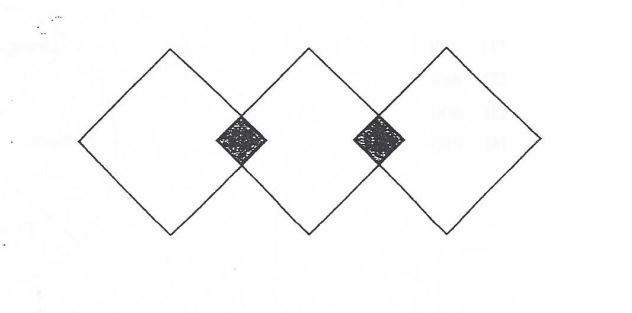# Question 1 of 52

The figure below shows 3 overlapping identical squares, with two overlapped area of the same size. If the area of each big square is 120cm$^2$ and the area of the whole figure is 300cm $^2$ , What is the area of each overlapped part?A
30cm$^2$
B
60cm$^2$
C
90cm$^2$
D
120cm$^2$
E
None of the above